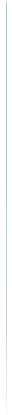Research Interests
Regression Analysis:
•  Identification of outliers
•  Robust regression
•  Principal components regression
•  Logistic regression

Statistical Computation:
•  Algorithm
•  Optimization techniques
•  Simulation

Customer relationship management:
•  Data mining

Marketing research:
•  Conjoint analysis
•  Survey methodology
PublicationsKim. B. Y. and Lee S. (2015). A cross-cultural study of body image perceptions between Korean and British university students. Fashion Business, 19(6), 14-27.Kim. B. Y. (2014). An empirical comparison of predictability of ranking-based and choice-based conjoint analysis. The Korean Journal of Applied Statistics, 27(5), 681-691.Kim. B. Y. (2014). New method for preference measurement in ranking-based conjoint analysis and its application to analysis of consumer preferences for perfume-for-women. The Korean Journal of Applied Statistics, 27.Kim, J., Yang, K. and Kim, B.Y. (2013). Online retailer reputation and consumer response: examining cross cultural differences, International Journal of Retail and Distribution Management, 41(9), 688-705.Kim, B.Y., Kim, J. and Kahn, Y. (2012). Analysis of consumer preferences for cosmetic essence-for-men via choice-based conjoint with new design of choice sets, The Korean Journal of Applied Statistics, 25(6), 987-997.Kim. B. Y. (2012), New design of choice sets for choice-based conjoint analysis, The Korean Journal of Applied Statistics, 25(5), 847-857.Kim. B. Y. (2011), A criterion for the selection of principal components in the robust principal component regression, The Korean Communications in Statistics, 18(6), 761-770.Kim. B. Y. and Shin, M. H. (2010), Procedure for the selection of principal components in principal components regression, The Korean Journal of Applied Statistics, 23(5), 967-975.Kim, B. Y. (2010), Algorithm for the L1-regression estimation with high breakdown point, The Korean Communications in Statistics, 17(4), 541-550.Kahng, M. W., Kim, B. Y., and Hong, J. H. (2010), Graphical regression and model assessment in logistic model,Journal of the Korean Data & Information Science Society, 21, 21-32.Son, O., Cheon, C. L., Kim, B. Y. et al (2010). ATHB12, an ABA-Inducible Homeodomain-Leucine Zipper(HD-Zip) Protein of Arabidopsis, Negatively Regulates the Growth of the Inflorescence Stem by Decreasing the Expression of a Gibberellin 20-Oxidase Gene, Plant & Cell Physiology, 51(9), 1537-1547.Kim, B. Y., Kahng, M. W., and Jang, H. W. (2009), Principal components logistic regression based on robust estimation, The Korean Journal of Applied Statistics, 22, 531-539.Kahng, M. W. and Kim, B. Y. (2009), Assessing the accuracy of outlier tests in nonlinear regression, The Korean Communications in Statistics, 16, 163-168.Kim, B. Y. and Kahng, M. W. (2008), Principal components regression in logistic model, The Korean Journal of Applied Statistics, 21, 571-580.Kahng, M. W., Kim, B. Y., and Jeon, J. Y. (2008), Three dimensional CERES plot in generalized linear models, The Korean Journal of Applied Statistics, 21, 169-176.Kim, B. Y., Kahng, M. W., and Choi, M. A. (2007), Algorithm for the robust estimation in logistic regression, The Korean Journal of Applied Statistics, 20, 551-559.Shin, Y. J., Kim, B. Y., and Hyun, Y. J. (2007), Conjoint analysis for the effects of cigarette warning label and packaging on intention to quit,Journal of Health and Social Affairs, 27(1), 27-51.Kim, B. Y. (2005), Conjoint analysis for the development of new cellular phone, Journal of the Korean Society for Quality Management, 33, 103-110.Kim, B. Y. (2005), V-mask type criterion for identification of outliers in logistic regression, The Korean Communications in Statistics, 12, 625-634.Kim, B. Y. andLee, S. Y. (2004), Identification of outliers in logistic regression, Journal of Natural Sciences, 15, 103-110.Kim, B. Y. and Oh, M. H. (2004), Identification of regression outliers based on clustering of LMS-residual plots, The Korean Communications in Statistics, 11, 485-494.Kim, B. Y. (2004), Resampling-based test of hypothesis in L1-regression, The Korean Communications in Statistics, 11, 643-655.Kim, B. Y. (2004), Conjoint analysis based on the Chebyshev estimation, with application to new product development of cellular phone, The Korean Journal of Applied Statistics, 17, 205-218.Kim, B. Y. and Jung, E. J. (2003), Robust estimation in the logistic regression model with hidden layer, Journal of Natural Sciences, 14, 133-140.Kim, B. Y. and Shin, K. H. (2002), A comparison of the response methods in conjoint analysis, Journal of Natural Sciences, 13, 127-133.Kim, B. Y. and Kim, H. Y. (2002), A hybrid algorithm for identifying multiple outliers in linear regression, The Korean Communications in Statistics, 9, 291-304.Kim, B. Y. and Shin, S. I. (2000), Partial residual plots based on robust estimators, Journal of Natural Sciences, 11, 145-153.Kim, B. Y. (2000), Algorithm for the constrained Chebyshev estimation in linear regression, The Korean Communications in Statistics, 7, 47-54.Kim, B. Y. and Park, H. S. (1999), Robust ridge estimation procedure for linear regression model, Journal of Natural Sciences, 10, 141-145.Kim, B. Y. and Lee H. Y. (1999), Updating techniques of orthogonal decomposition, Journal of Natural Sciences, 10, 135-140.Kim, B. Y. (1998), Constrained L1-estimation in Linear Regression, The Korean Communications in Statistics, 5, 581-590.Kim, B. Y. and Kim S. B. (1997), Improvements in computational efficiency and accuracy of an algorithm for the identification of regression outliers, Journal of Natural Sciences, 8, 135-142.Kim, B. Y. (1996), Improved procedure for least median of squares estimation and application to identification of multiple outliers, Yonsei Economic Studies, 3(2), 185-203.Kim, B. Y. (1996), L -estimation based algorithm for the least median of squares estimator, The Korean Communications in Statistics, 3(2), 299-307.Kim, B. Y. (1995), Computational aspects of the least median of squares estimation, Journal of Natural Sciences, 6, 129-138.Kim, B. Y. (1995), On the robustness of L1-estimator in linear regression models, The Korean Communications in Statistics, 2, 277-287.Kim, B. Y. (1994), Identification of multiple outliers based on the robust regression, Journal of Natural Sciences, 5, 129-138.Kim, B. Y. (1993), A goodness-of-fit test for the exponentiality with censored samples, The Korean Journal of Applied Statistics, 6, 289-302.Kim, B. Y. (1991), A goodness-of-fit test for the exponential distribution with unknown parameters, The Korean Journal of Applied Statistics, 4, 157-170.Sherali, H., Skarpness, B., and Kim, B. Y. (1988), An assumption-free convergence analysis for a perturbation of the scaling algorithm for linear programs, with application to the L1-estimation problem, Naval Research Logistics, 35, 473-492.Kim, B. Y. (1987), A robust estimation procedure for the linear regression model, The Journal of Korean Statistical Society, 16, 80-91.
Working Papers